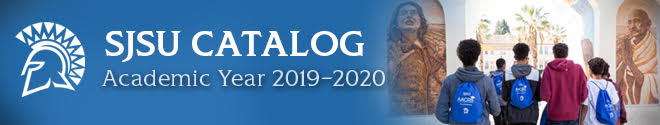# Info.sjsu.eduIndex: A B C D E F G H I J K L M N O P Q R S T U V W X Y Z Search Keyword

### Regression Theory and Methods

Description
Simple linear regression, multiple regression, indicator variables, influence diagnostics, transformations, assumption analysis, generalized linear models, nonlinear regression, CART, hypothesis testing, confidence and prediction intervals, variable selection and model building. Prerequisites: Math 129A, and either Math 161A or Math 164 (each with grade"B" or better), Math 163, Math 267A. Math 163 and/or Math 267A may be taken concurrently.